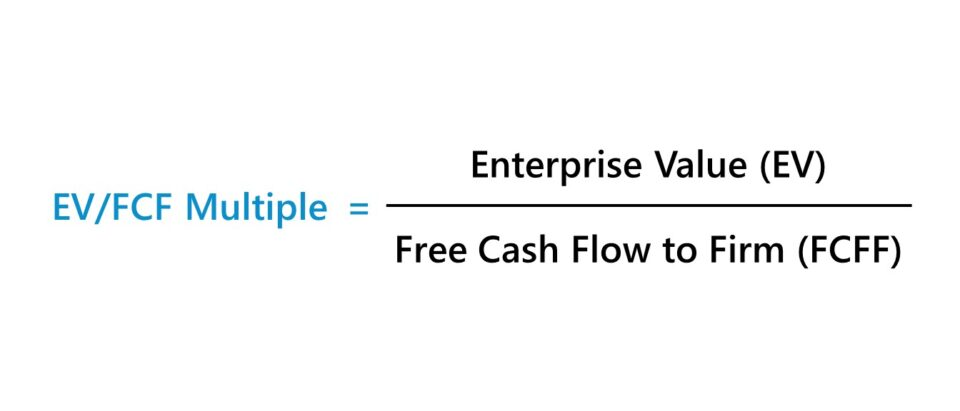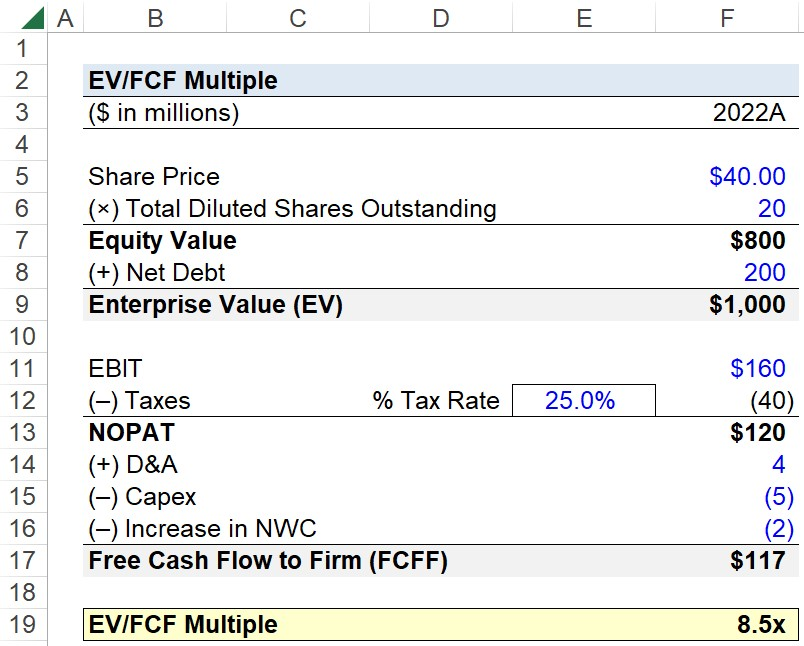Welcome to Wall Street Prep! Use code at checkout for 15% off.# EV/FCF Multiple

Guide to Understanding EV/FCF## How to Calculate EV/FCF?

The EV/FCF multiple is the ratio between enterprise value and free cash flow.

• Enterprise Value (EV): The enterprise value measures the value of a company’s operations from the perspective of all capital providers, such as debt lenders, common shareholders, and preferred stockholders.
• Free Cash Flow (FCF): Since the numerator is enterprise value, an unlevered metric, the free cash flow to firm (FCFF) is the appropriate metric to use. FCFF, or “unlevered free cash flow,” is the FCF generated by a company’s core operations that belongs to all stakeholders.

To ensure consistency in the valuation multiple – with respect to the group(s) of capital providers represented – it is necessary for the cash flow metric to be free cash flow to firm (FCFF). Otherwise, there is a mismatch in stakeholder representation in the numerator and denominator.

For instance, the free cash flow to equity (FCFE) metric reflects the cash flows attributable to only equity holders, i.e. post-interest and mandatory debt repayments.

Thus, the corresponding measure of value in the numerator should be equity value (or “market cap”), instead of enterprise value.

## EV/FCF Formula

The formula to calculate the EV/FCF multiple is as follows.

EV/FCF = Enterprise Value ÷ Free Cash Flow to Firm (FCFF)

The two inputs are calculated using the following formulas.

Enterprise Value (TEV) = Equity Value + Net Debt + Preferred Stock + Minority Interest
Free Cash Flow to Firm (FCFF) = NOPAT + D&A  Increase in NWC  Capex

## How to Interpret EV/FCF?

The EV/FCF multiple answers the question, “For each dollar of unlevered free cash flow (FCFF) generated by a company, how much are investors currently willing to pay?”

Thus, the higher the EV/FCF ratio, the greater the premium attached to a dollar of a company’s unlevered free cash flow (and vice versa).

However, the downside to using the EV/FCF multiple is that a company’s free cash flow to firm (FCFF) tends to fluctuate substantially over time.

Based on the timing of certain events, such as periodic capital expenditures and cyclicality (or seasonality) in working capital levels, the EV/FCF multiple can become distorted. In effect, the ratio tends to be less practical for comparisons, which is the core premise of relative valuation.

Furthermore, the calculation of free cash flow to firm (FCFF) contains more discretionary decisions with less standardization among practitioners, even more so than non-GAAP metrics like EBITDA (and adjusted EBITDA).

For that reason, valuation multiples such as EV/EBITDA and EV/EBIT are far more prevalent in usage.

## What’s the Difference Between EV/FCF vs. Unlevered FCF Yield?

The EV/FCF valuation multiple is the inverse of the unlevered FCF yield metric.

Unlevered FCF Yield (%) = Free Cash Flow to Firm (FCFF) ÷ Enterprise Value

The unlevered FCF yield metric reflects the cash remaining that is attributable to all providers of capital (e.g. debt and equity), expressed in percentage form.

## EV/FCF Calculator – Excel Template

We’ll now move on to a modeling exercise, which you can access by filling out the form below.Submitting...

## Step 1. Enterprise Value Calculation (EV)

Suppose you’re tasked with calculating the EV/FCF multiple of a company given the following set of assumptions.

By multiplying the share price by the total diluted shares outstanding, the equity value of the company is \$800 million.

• Equity Value = \$40.00 × 20 million = \$800 million

To move from equity value to enterprise value (EV), we’ll add net debt, resulting in an enterprise value of \$1 billion.

• Enterprise Value (EV) = \$800 million + \$200 million = \$1 billion

## Step 2. Free Cash Flow to Firm Calculation (FCFF)

In the next step, we’ll calculate our company’s free cash flow to firm (FCFF) using the following set of assumptions.

The starting point of the FCFF is NOPAT, which we’ll calculate by tax-affecting EBIT.

• NOPAT = \$160 million – (\$160 million × 25%) = \$120 million

From NOPAT, we’ll add D&A, subtract Capex, and subtract an increase in NWC to arrive at our company’s free cash flow to firm (FCFF).

• Free Cash Flow to Firm (FCFF) = \$120 million + \$4 million – \$5 million – \$2 million = \$117 million

## Step 3. EV/FCF Calculation Example

With our two inputs calculated, the final step is to divide our company’s enterprise value by its free cash flow to firm (FCFF), which returns an EV/FCF multiple of 8.5x.

• EV/FCF = \$1 billion ÷ \$117 million = 8.5xStep-by-Step Online Course

### Everything You Need To Master Financial Modeling

Enroll in The Premium Package: Learn Financial Statement Modeling, DCF, M&A, LBO and Comps. The same training program used at top investment banks.